Disclaimer: This is an example of a student written essay.

Any scientific information contained within this essay should not be treated as fact, this content is to be used for educational purposes only and may contain factual inaccuracies or be out of date.

# Power Sytems Load Flow Analysis

 ✅ Paper Type: Free Essay ✅ Subject: Engineering ✅ Wordcount: 5337 words ✅ Published: 18th May 2020

### ABSTRACT

Study of power flow is the investigation of a scheme that is subjected to imbalance demands under steady state operation in which the system operates. Power flow examination is the basis for analysing and planning energy systems. For planning, operation, future extension and power exchange between utilities, they are vital. The power flow test information is to discover voltage magnitude and phase angle and real and reactive power flow in the transmission line.

If you need assistance with writing your essay, our professional essay writing service is here to help!

Iterative methods are used in this inquiry because systematic approach is known to address the issues of power flow. Power flow testing takes time to execute by hand. For this project, the NEWTON RAPHSON method is used. The objective of this project is to construct a power flow program based on the Newton Raphson method that will make it easier to investigate the power flow issues. MATLAB software was used for programming.

Library Session- Research, Referencing and Report writing:-

In previous semester we had done library session and learn how to research , referencing and how to write report.

Matlab:-

MATLAB is an interactive system and programming language for general scientific and technical computation. In previous semester we are studying basic about the MATLAB coding. And also learn where to type commands, how to execute commands, how to see output, etc.

m-File: Script  File

m-File or script file is a simple text where you can place MATLAB commands. All m-file names must end with the extension ‘.m’. If you create a new m-file with the same name as an existing m-file, MATLAB will choose the one which appears first in the path order.

m-File: Functions File

Functions are program routines, usually implemented in M-files, that accept input arguments and return output arguments. They operate on variables within their own workspace. This workspace is separate from the workspace you access at the MATLAB command prompt. Every M-File functions have memory, different from the the MATLAB base workspace, in which it operates.

Example:-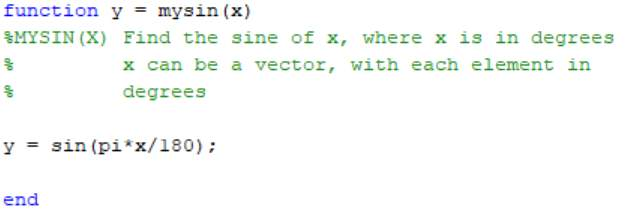MATLAB PLOTS AND INPUT/OUTPUT

A = [1 2 3 4 6 4 3 4 5]

A = 1×9

1     2     3     4     6     4     3     4     5

B= A+ 2

B = 1×9

3     4     5     6     8     6     5     6     7

plot(B)

grid on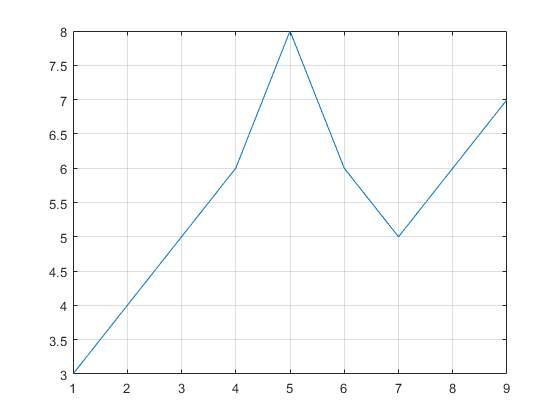Electrical  measurement Lab:

In previous semester we learn how to measure electrical quantities, learn properties of electrical meters, power supplies and learn use of oscilloscope.

Standard resistor values and colour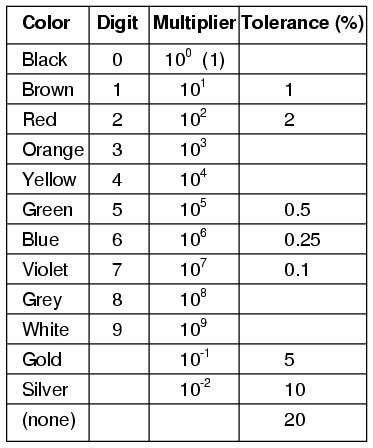With the help of standard resistors and colour code we can find the values of resistor 4-band and 5-band code.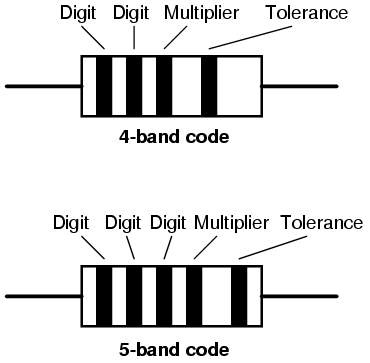Also solve the circuit with the help of voltage divider and current divider rule and also find the current and voltage through each components.

Oscilloscope:

Oscilloscope is a device which used to display and analyse the waveform of electronic signals. In previous semester we use oscilloscope for the generate signal of voltage. On the y-axis voltage and y-axis for seconds.

Example:

Voltage signal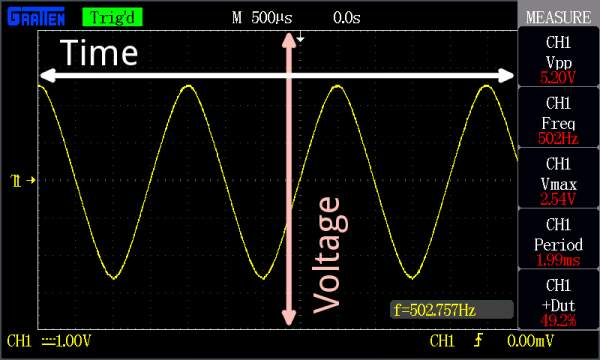CV Writing / Job Search / Interviews

In this session we learn how to make effective  CV(resume) and also after making you can check CV from student support Team and you can search jobs from career and employment solution and also teach  how to crack interviews.

Load Flow study is the analysis of power system in normal steady state condition. Load Flow Study is determination of

1. Voltage
2. Current
3. Active power
4. Reactive power

1. The magnitude of the bus voltage stays near the rated value.
2. Generation works within defined boundaries of actual and reactive power.
3. Transmission line and transformer are not overloaded.

Bus Classification: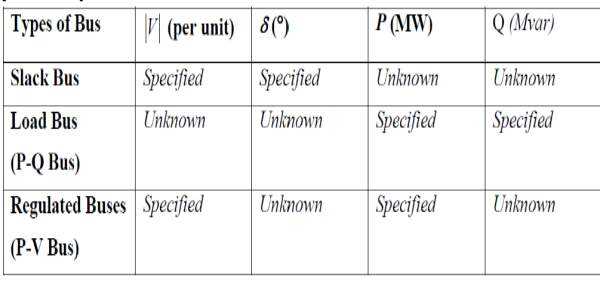Load flow can be solved by two techniques:

1. Gauss-Seidel Method
2. Newton-Raphson Method

Among this method I used Newton-Raphson method because this strategy is famous because of its intermingling with a less cycles.

1). Gauss-Seidel Method-

Gauss-Seidel  method  is an iterative method used to solve a linear system of equations and is similar to the Jacobi Method. It can be applied to any matrix with non-zero elements on the diagonals, convergence is only guaranteed if the matrix is either diagonally dominant, or symmetric and positive definite.

2). Newton-Raphson Method-

The newton-Raphson method of solution enables us to replace the non-linear of intensity conditions with a direct set, streamlining the arrangement. We review the iterative arrangement of non-linear synchronous conditions by the Newton-Raphson strategy, and that after that apply the method to power flow.

In this semester we are studied about the Nodal analysis and power relation which is required for the load flow study.

Nodal Analysis of System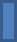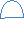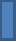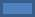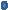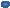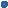1

2

3

${Y}_{23}$

${Y}_{12}$

${Y}_{13}$

${Y}_{10}$

${Y}_{130}$

${Y}_{20}$

Using the KCL equations are

${I}_{1}={y}_{10}{v}_{1}+{y}_{12}\left({v}_{1}–{v}_{2}\right)+{y}_{13}\left({v}_{1}–{v}_{2}\right)$

${I}_{2}={y}_{20}{v}_{2}+{y}_{12}\left({v}_{2}–{v}_{1}\right)+{y}_{13}\left({v}_{2}–{v}_{3}\right)$

${I}_{3}={y}_{30}{v}_{3}+{y}_{13}\left({v}_{3}–{v}_{1}\right)+{y}_{23}\left({v}_{3}–{v}_{2}\right)$

So that we have,

${I}_{1}={\left(y}_{10}$

+

)

+ ( $–{y}_{12}$

)

+ ( $–{y}_{13}$

)

${I}_{2}={\left(y}_{20}$

+

)

+ ( $–{y}_{12}$

)

+ ( $–{y}_{23}$

)

${I}_{3}={\left(y}_{30}$

+

)

+ ( $–{y}_{13}$

)

+ ( $–{y}_{23}$

)

So that we can write like

${Y}_{11}={y}_{10}$

+

${Y}_{22}={y}_{20}$

+

${Y}_{33}={y}_{30}$

+

And

${Y}_{12}=–{y}_{12}$

${Y}_{13}=$ $–{y}_{13}$

${Y}_{23}=–{y}_{23}$

From this equation convert this into matrix form

$\begin{array}{c}{I}_{1}\\ {I}_{2}\\ {I}_{3}\end{array}$

= $\begin{array}{ccc}{Y}_{11}& {Y}_{12}& {Y}_{13}\\ {Y}_{12}& {Y}_{22}& {Y}_{23}\\ {Y}_{13}& {Y}_{23}& {Y}_{33}\end{array}$

* $\begin{array}{c}{v}_{1}\\ {v}_{2}\\ {v}_{3}\end{array}$

Simply we can write

${I}_{\mathit{BUS}}={Y}_{\mathit{BUS}}{V}_{\mathit{BUS}}$

POWER RELATION

With the help of power relation we will get the real power and imaginary power equation and this will we can use in the power flow study.

We have

${I}_{\mathit{BUS}}={Y}_{\mathit{BUS}}{V}_{\mathit{BUS}}$

We can redefine and write as

${I}_{\mathit{BUS}}=A+\mathit{jC}$

,  A= real part and C= imaginary part

${Y}_{\mathit{BUS}}=D+\mathit{jF}$

, D= real part and F= imaginary part

${V}_{\mathit{BUS}}=G–\mathit{jB}$

, G= real part and B= imaginary part

For the N number of element we can write

${I}_{i}={A}_{i}+j{C}_{i}$

,                         i= 1,2,3,……,N

${Y}_{\mathit{ii}}={G}_{\mathit{ii}}–j{B}_{\mathit{ii}}$

,                    i= 1,2,3,……,N

${V}_{i}={D}_{i}+j{F}_{i}$

,                        i= 1,2,3,……,N

LET the Bus power be

${S}_{i}={P}_{i}–j{Q}_{i}={V}_{i}^{*}{I}_{i}$

And we know the equation

${S}_{i}=\sum _{k=1}^{N}{{V}_{i}^{*}V}_{\mathit{ik}}{V}_{k}$

Substitute the equation

For the real part we can write the real power

${P}_{\mathbit{i}}\mathbit{=}\sum _{\mathbit{k}\mathbit{=}\mathbit{1}}^{\mathbit{N}}{\mathbit{D}}_{\mathbit{i}}{\mathbit{G}}_{\mathbit{ik}}{\mathbit{D}}_{\mathbit{k}}\mathbit{+}{\mathbit{D}}_{\mathbit{i}}{\mathbit{B}}_{\mathbit{ik}}{\mathbit{F}}_{\mathbit{k}}\mathbit{–}{\mathbit{F}}_{\mathbit{i}}{\mathbit{B}}_{\mathbit{ik}}{\mathbit{D}}_{\mathbit{k}}\mathbit{+}{\mathbit{F}}_{\mathbit{i}}{\mathbit{G}}_{\mathbit{ik}}{\mathbit{F}}_{\mathbit{k}}$

and

${Q}_{\mathbit{i}}\mathbit{=}\sum _{\mathbit{k}\mathbit{=}\mathbit{1}}^{\mathbit{N}}{\mathbit{D}}_{\mathbit{i}}{\mathbit{B}}_{\mathbit{ik}}{\mathbit{D}}_{\mathbit{k}}\mathbit{–}{\mathbit{D}}_{\mathbit{i}}{\mathbit{G}}_{\mathbit{ik}}{\mathbit{F}}_{\mathbit{k}}\mathbit{+}{\mathbit{F}}_{\mathbit{i}}{\mathbit{G}}_{\mathbit{ik}}{\mathbit{D}}_{\mathbit{k}}\mathbit{+}{\mathbit{F}}_{\mathbit{i}}{\mathbit{B}}_{\mathbit{ik}}{\mathbit{F}}_{\mathbit{k}}$

Write the equation for ${I}_{i}$

and ${V}_{i}$

So        ${I}_{i}=$ $\frac{{P}_{i}–j{Q}_{i}}{{V}_{i}^{*}}$

$\left[K\ne i\right]$

TIME PLAN AND BUDGET

Conclusion:

• The objective of this project to learn about load flow by using Gauss-Seidel or Newton-Raphson Method and learn MATLAB programming and simulation for load flow analysis using MATLAB.
• In Previous Semester we studied about basic coding of MATLAB, Electrical measurement and how to make cv and technique to crack  interviews.

REFERENCES

View all

## DMCA / Removal Request

If you are the original writer of this essay and no longer wish to have your work published on UKEssays.com then please:

Related Services

Prices from

£126

Approximate costs for:

• 1000 words
• 7 day deliveryHumanity University

Dedicated to your worth and value as a human being!

Related Lectures